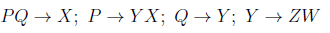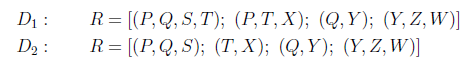# GATE | GATE CS 2021 | Set 1 | Question 43

• Last Updated : 24 May, 2021

Consider the relation R(P,Q,S,T,X,Y,Z,W) with the following functional dependencies.Consider the decomposition of the relation R into the constituent relations according to the following two decomposition schemes.Which one of the following options is correct?
(A) D1 is a lossless decomposition, but D2 is a lossy decomposition
(B) D1 is a lossy decomposition, but D2 is a lossless decomposition
(C) Both D1 and D2 are lossless decompositions
(D) Both D1 and D2 are lossy decompositions

Explanation: Lossless-Join Decomposition:
Lossless-Join Decomposition:
Decomposition of R into R1, R2, R3, R4 is a lossless-join decomposition if at least one of the following functional dependencies are in F+ (Closure of functional dependencies):

```R1 ∩ R2 → R1
OR
R1 ∩ R2 → R2```

For decomposition D1:

R1(PQST)

R2(PTX)

R3(QY)

R4(YZW)

R1 ∩ R2 = (PT)+ = PTYXZW , it is a super key, so we can merge R1 and R2.

combined table T1 is PQSTX

similarly,

R3 ∩ R4 =(Y)+ = YZW, it is a super key,  so we can merge R3 and R4.

another combined table T2 is QYZW.

now, Q is common in both T1 and T2.

T1 ∩ T2 = Q+ = QYZW, it is a super key, so we can merge T1 and T2.

after combining, we get original table PQSTXYZW,

Hence D1 is lossless join decomposition.

For decomposition D2:

R1(PQS)

R2(TX)

R3(QY)

R4(YZW)

since R2 has no common attributes as the primary key, so R2 cannot be merge with any other table,

Hence D2 is lossy decomposition.

My Personal Notes arrow_drop_up
Recommended Articles
Page :